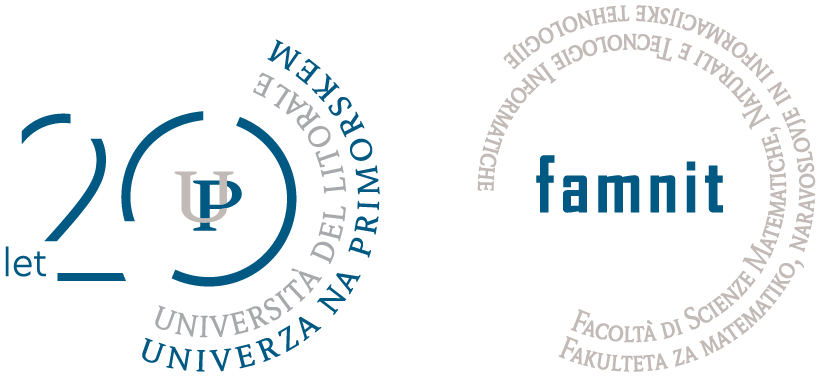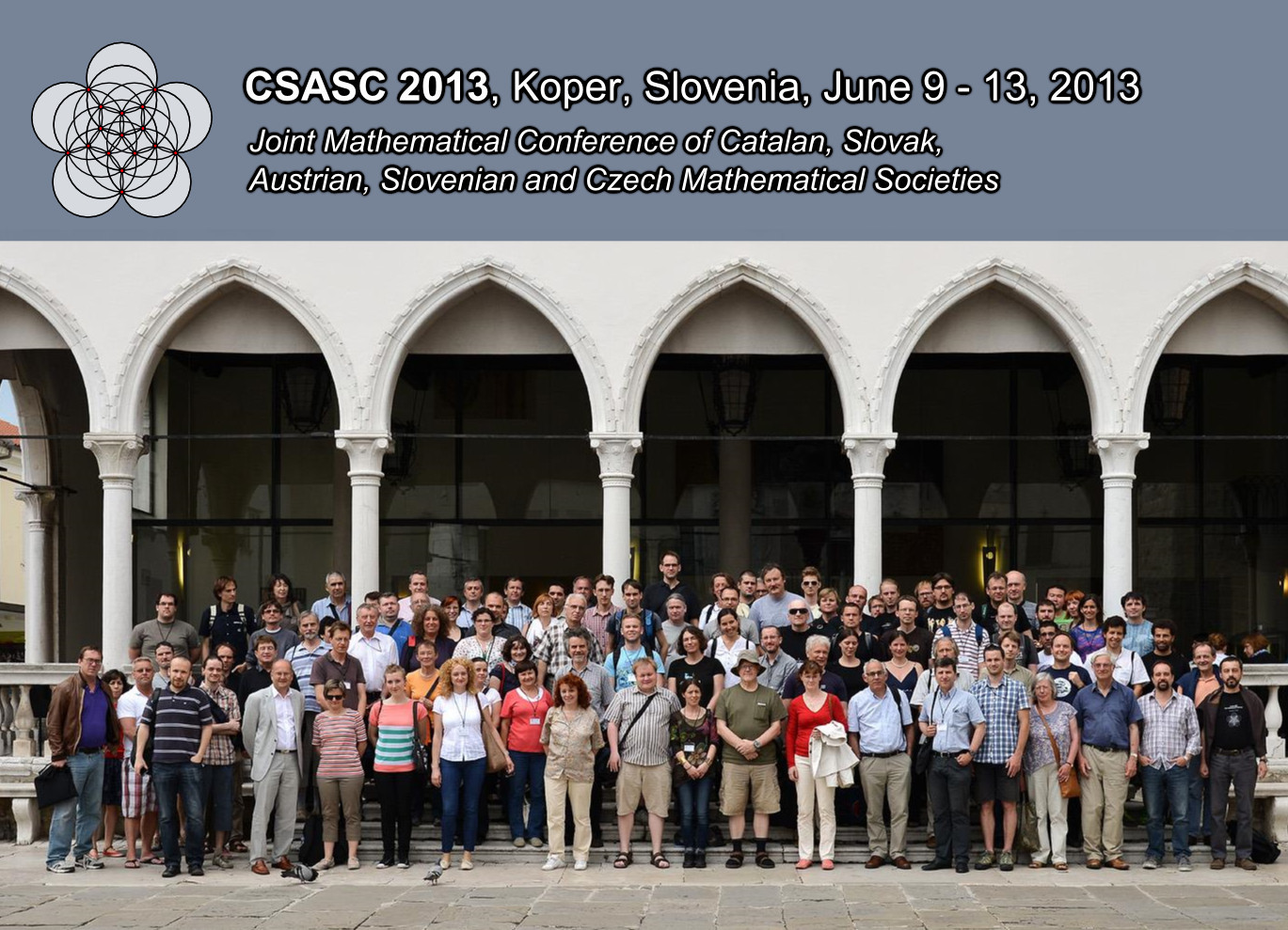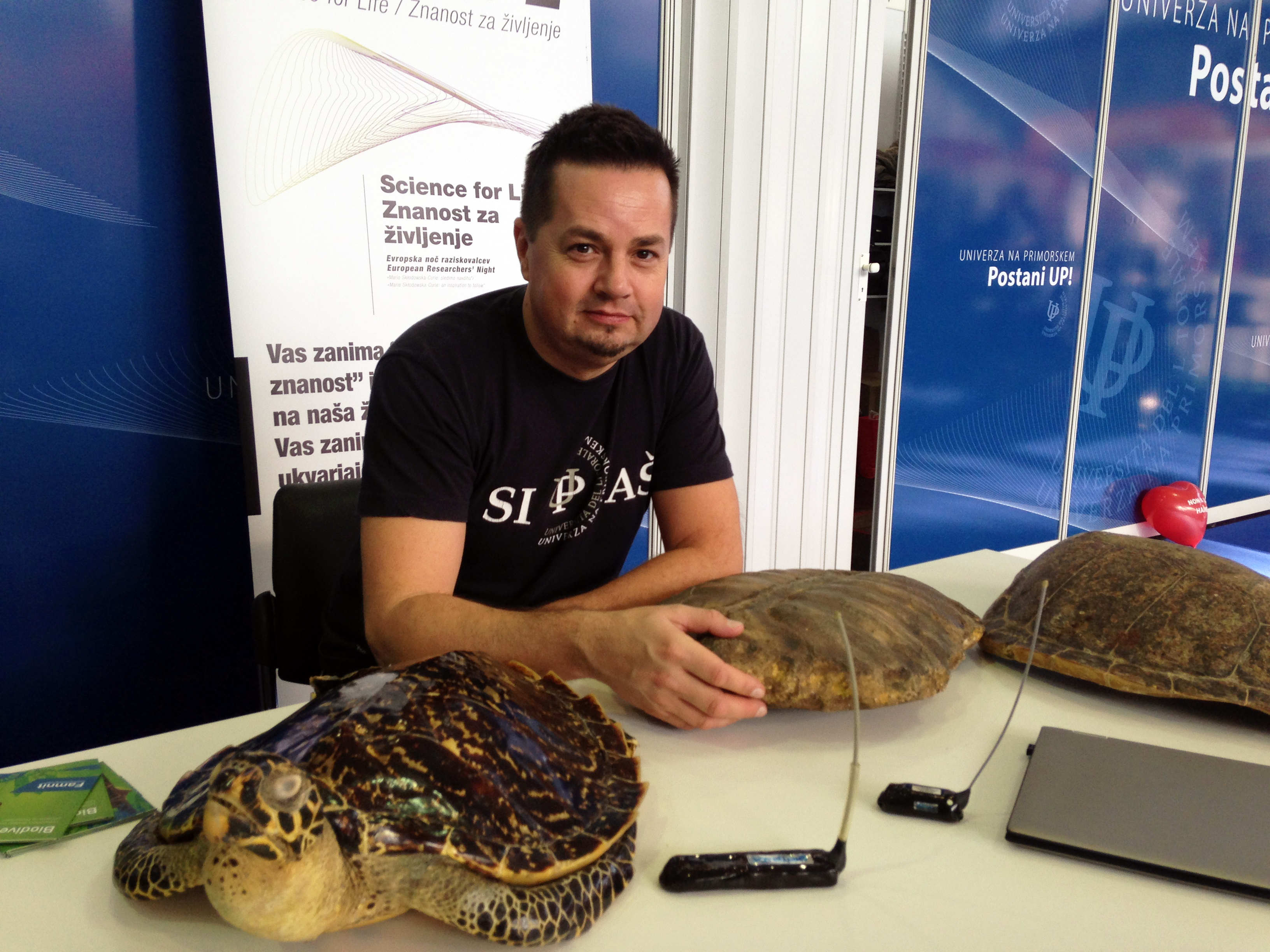Univerza na Primorskem Fakulteta za matematiko, naravoslovje in informacijske tehnologijeSI | EN# Raziskovalni matematični seminar - Arhiv

 2023 2022 2021 2020 2019 2018 2017 2016 2015 2014 2013 2012 2011 2010
 1 2 3 4 5 6 7 8 9 10 11 12

28.03.2012 Lecturer: dr. Gábor Gévay (University of Szeged, Hungary)

Title: Geometric (nk) configurations

Abstract: In the simplest case, a geometric (nk) configuration is a set of n points and n lines such that each of the points is incident with precisely k of the lines and each of the lines is incident with precisely k of the points. Instead of lines, the second subset may consist of planes, hyperplanes, circles, ellipses, etc. We discuss some construction principles, and review some recently discovered classes of such configurations. We also formulate an incidence conjecture concerning a spatial (1004) point-line configuration.

26.03.2012 Lecturer: dr. Marcin Anholcer (Poznan Univeristy of Economics, Poland)

Title: Group irregularity strength of graphs.

Abstract: We investigate the \textit{group irregularity strength} ($s_\mathcal{G}(G)$) of graphs, i.e. the smallest value of $s$ such that taking any Abelian group $\mathcal{G}$ of order $s$, there exists a function $f:E(G)\rightarrow \mathcal{G}$ such that the sums of edge labels in every vertex are distinct. We prove that for any connected graph $G$ of order at least $3$, $s_\mathcal{G} (G)=n$ if $n\neq 4k+2$ and $s_\mathcal{G} (G)\leq n+1$ otherwise, except the case of some infinite family of stars.

19.03.2012 Lecturer: dr. Oliver Schaudt (Universität zu Köln, Germany).

Title: The Price of Connectivity for Vertex Cover

Abstract: We study a graph parameter called the Price of Connectivity for Vertex Cover (PoC). It was recently introduced by Cardinal et al. and is defined as the ratio of the minimum size of a connected vertex cover and the minimum size of a vertex cover.We prove some structural results for the PoC. These results concern PoC-critical, PoC-perfect and PoC-near-perfect graphs.
Joint work with E. Camby, J. Cardinal and S. Fiorini.

12.03.2012 Lecturer: dr. Istvan Kovacs

Title: The isomorphism problem for rose window graphs

Abstract: For an integer n ≥ 3, and a, r ∈ {1, 2, ..., n − 1} with r ≠ n/2, the rose window
graph Rn (a, r) is the graph Γ, where V (Γ) = {Ai , Bi | i ∈ {0, 1, ..., n − 1}}, and E(Γ) consists of four types of edges as for every i ∈ {0, 1, ..., n − 1}: {Ai , Ai+1 }, {Ai , Bi }, {Ai+a , Bi } and {Bi , Bi+r},
where addition is taken modulo n. In this talk we consider the following problem:
given a rose window graph Rn (a, r), ﬁnd all pairs (b, s) ∈ {1, 2, ..., n−1}2 for which
Rn (b, s) is isomorphic to Rn (a, r). We present a solution using a permutation
group theoretical approach.
This is a joint work with E. Dobson and Š. Miklavič.

05.03.2012 Lecturer: dr. Marko Orel

Title: The mathematics in computed tomography (CT)

Abstract: In 1979, the Nobel Prize for Medicine and Physiology was awarded jointly to Allan
McLeod Cormack and Godfrey Newbold Hounsfield, the two pioneering scientistengineers
primarily responsible for the development, in the 1960s and early 1970s,
of computerized axial tomography, popularly known as the CAT or CT scan.
At the seminar I will present the mathematics involved in computed tomography.